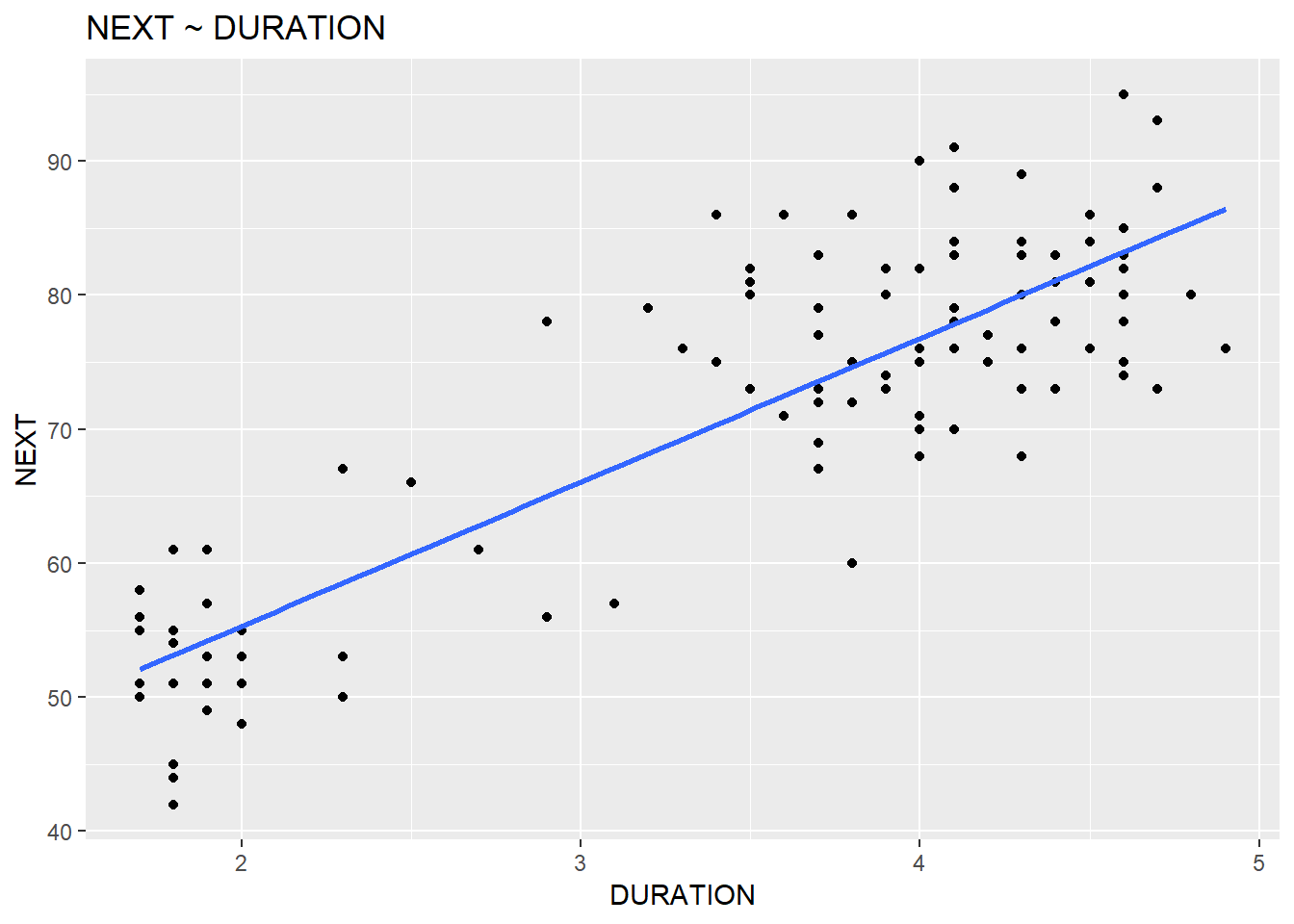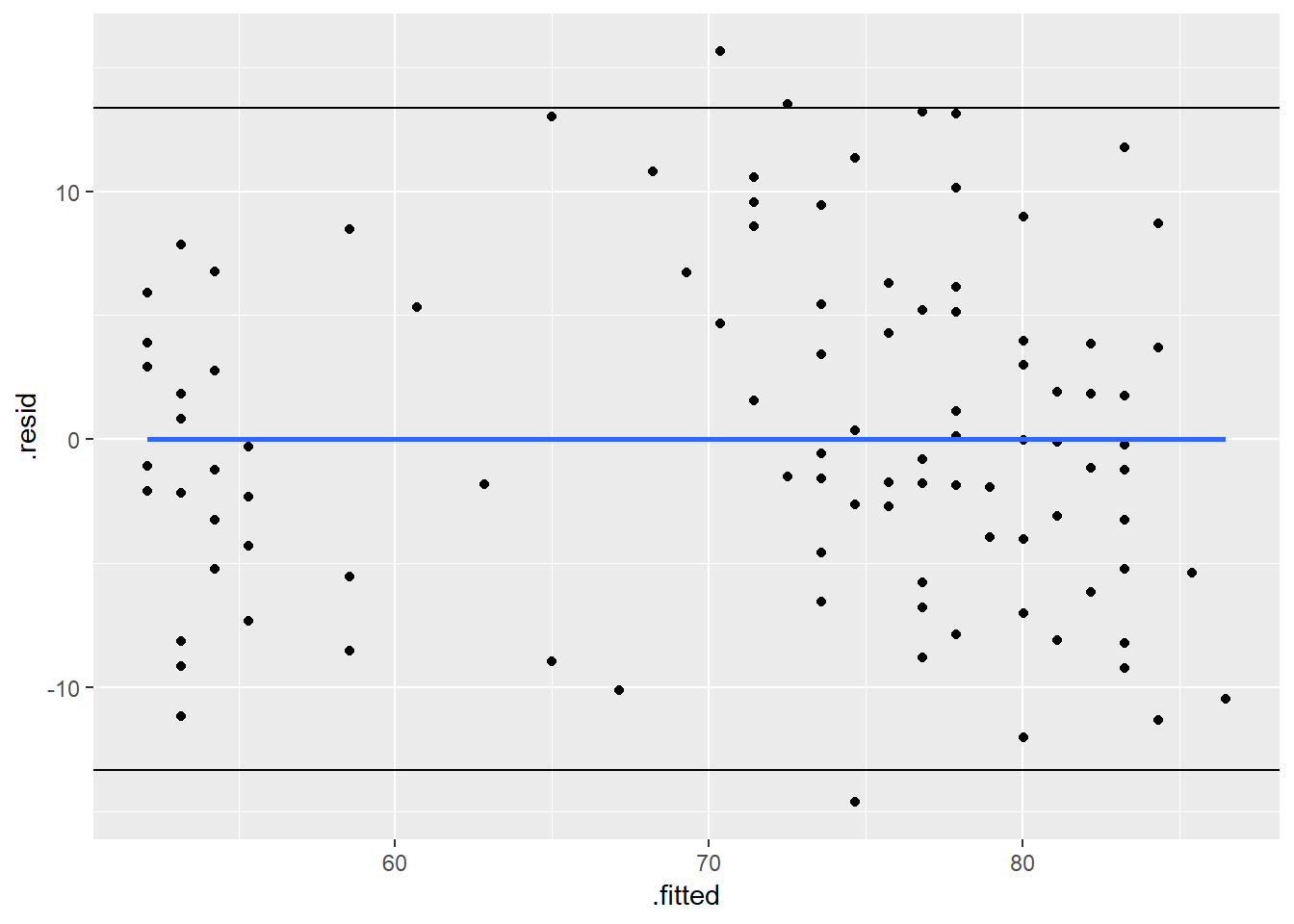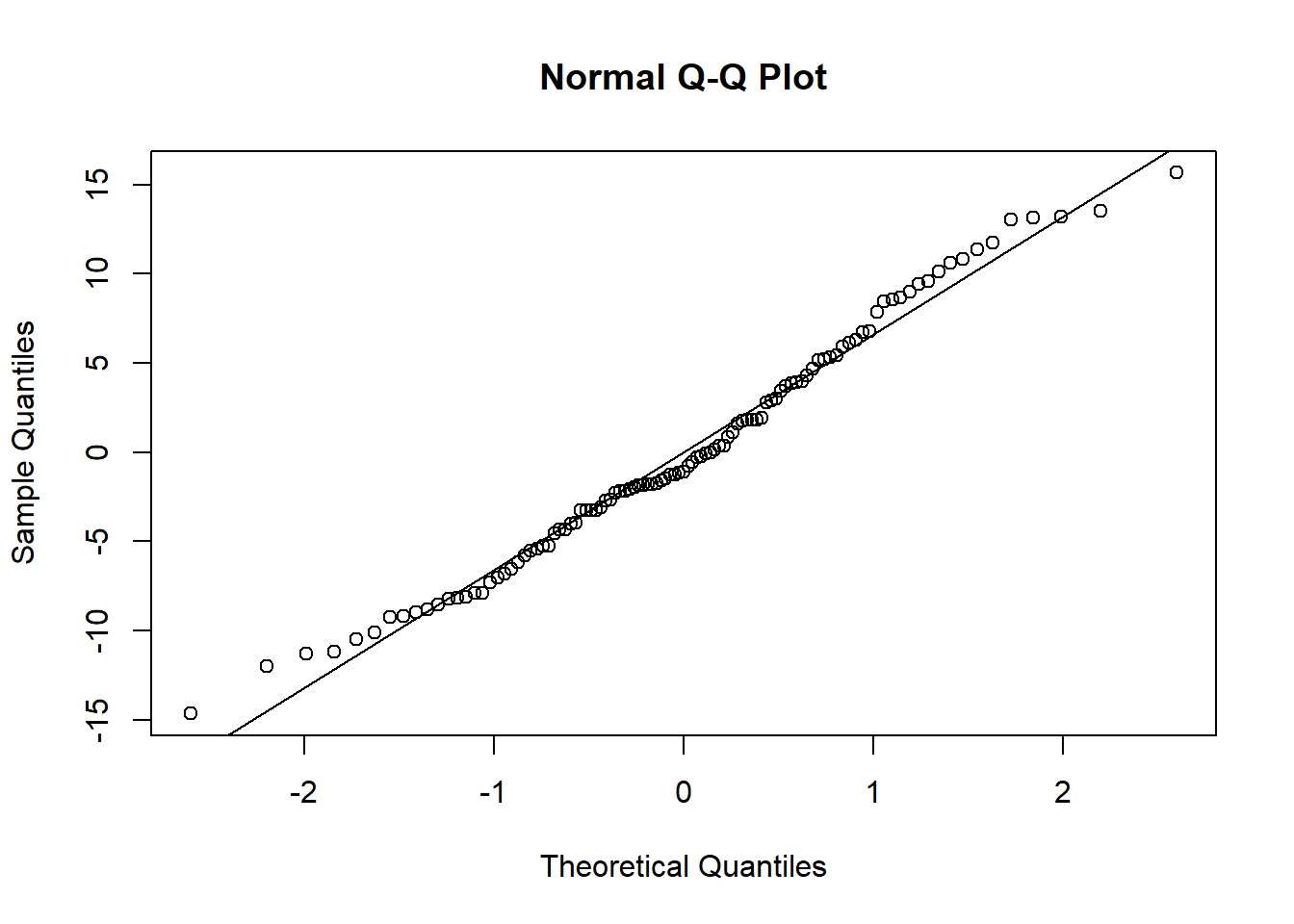# Simple Linear Regression

## Background: Ordinary Least Squares

The population regression line $$\mu_Y = E(Y) = X \beta$$ summarizes the trend in the population between the predictors and the mean of the responses. The individual responses vary from the population regression, $$y = X \beta + \epsilon$$. Estimate the population model as $$\hat{y} = X \hat{\beta}$$. Ordinary least squares (OLS) minimizes the sum of squared errors $$(y - \hat{y_i})^2$$ to determine $$\hat{\beta}$$.

The OLS estimator is consistent when the regressors are independent. The OLS estimator is the minimum-variance mean-unbiased estimator when the residuals $$\epsilon_i = y_i - \hat{y}_i$$ are also equal (homoscedastic). The OLS estimator is the Best Linear Unbiased Estimator (BLUE) when the errors are also normally distributed. Taken together, the OLS model is BLUE if the errors $$\epsilon_i$$ are independent normal random variables with mean zero and constant variance $$\sigma^2$$. Recall these conditions with the LINE pneumonic: Linear, Independent, Normal, and Equal.

Linearity. The explanatory variables are each linearly related to the response variable. I.e., $$E(\epsilon_i | X_i) = 0$$. A residuals vs fits plot $$(\epsilon \sim \hat{Y})$$ should bounce randomly around 0. An observed vs fits plot $$(Y \sim \hat{Y})$$ should be symmetric along the 45-degree line. Each $$(Y \sim X_i )$$ plot should have correlation $$\rho \sim 1$$. For simple linear regression, each residual plot $$(\epsilon \sim X_i)$$ should exhibit no pattern. If the linearity condition fails, change the functional form of the model with non-linear transformations of the explanatory variables.

Independence. The residuals are unrelated to the response variable (no multicollinearity). A residuals vs fits plot $$(\epsilon \sim \hat{Y})$$ should have correlation $$\rho \sim 0$$. All tests and intervals are sensitive to this condition.

Normality. The residuals are distributed nearly normally. A normal probability plot or a normal quantile plot should have values near the line with no bow-shaped deviated. A histogram should be normally distributed. A residuals vs fits plot $$(\epsilon \sim \hat{Y})$$ should be randomly scattered around 0. Sometimes the normality check fails when linearity assumption does not hold. Parameter estimation is not sensitive to this condition, but prediction intervals are.

Equal Variances. The variance of the residuals is constant (homoscedastic). The residuals should be the same size at both low and high values of the response variable. A residuals vs fits plot $$(\epsilon \sim \hat{Y})$$ should have random scatter in a band of constant width around 0, and no fan shape at the low and high ends. All tests and intervals are sensitive to this condition.

# Simple Linear Regression

Simple linear regression (SLR) is the simple case of OLS with a single explanatory variable. SLR models the mean response to a single predictor as $$y = E(Y) = \beta_0 + \beta_1 x$$. Any particular response is the mean response plus random variation: $$y_i = E (Y_i ) = \beta_0 + \beta_1 x + \epsilon_i$$. The model presumes a linear relationship between $$y$$ and $$x$$, and the errors $$\epsilon_i$$ are independent normal random variables with mean zero and constant variance $$\sigma^2$$. Estimate the population parameters, $$\beta_0$$, $$\beta_1$$, and $$\sigma^2$$ from a random sample. Denote the regression line fitted to the random sample as $$\hat{y} = \hat{\beta}_0 + \hat{\beta}_1 x$$.

SLR fits the model $$y = \beta_0 + \beta_1 x + \epsilon$$ so that $$y | x \sim N(\beta_0 + \beta_1 x, \sigma^2)$$. SLR minimizes the sum of squared prediction errors $$SSE = \sum_{i=1}^n {(y_i - \hat{y}_i)^2}$$ in the fitted equation $$\hat{y} = \hat{\beta}_0 + \hat{\beta}_1 x$$. In other words, SLR minimizes $$\sum_{i=1}^n{(y_i - (\hat{\beta}_0 + \hat{\beta}_1 x_i))^2}$$. SLR estimates parameters $$\beta_0$$ and $$\beta_1$$ with $$\hat{\beta}_0$$ and $$\hat{\beta}_1$$, and the population variance $$\sigma^2$$ with $$\hat{\sigma}^2$$.

# Parameter Estimation

Minimize the sum of squared residuals $$\sum_{i=1}^n{(y_i - (\hat{\beta}_0 + \hat{\beta}_1 x_i))^2}$$^2 by applying the first order conditions, $$\frac{{\delta SSE}}{{\delta \hat{\beta}_0}} = 0$$ and $$\frac{{\delta SSE}}{{\delta \hat{\beta}_1}} = 0$$. $$\hat{\beta}_1 = \frac{\sum_{i=1}^n(x_i - \bar{x})(y_i - \bar{y})}{\sum_{i=1}^n (x_i - \bar{x})^2} = \frac{SS_{xy}}{SS_x}$$ where $$SS$$ means the sum of squared deviations from the mean. Dividing the numerator and denominator by $$n$$ changes the ratio to the covariance $$s_{xy}^2$$ and variance $$s_x^2$$.

$$\hat{\beta}_1 = \frac{{s_{xy}^2}}{{s_x^2}}$$ $$\hat{\beta}_0= \hat{y} - \hat{\beta}_1 \bar{x}$$

By substituting the parameter estimators back into the regression equation and letting $$x = \bar{x}$$, it is apparent that the regression line The regression line passes through $$(\bar{x}, \bar{y})$$.

The mean square error estimates the common variance around the population regression line.

$$MSE = \hat{\sigma}^2 = \frac{\sum_{i=1}^n{(y_i - \hat{y}_i)^2}}{n-2}$$ The square root is the residual standard error.

## Parameter Estimation Example

The time (TIME) until the next eruption at Old Faithful Geyser is linearly related to the duration (DURATION) of the prior eruption. Using a data sample1 Data from PSU STAT 414, estimate the population parameters $$\beta_0$$, $$\beta_1$$, and $$\sigma$$ in the relationship TIME ~ DURATION.

The data consists of $$n = 107$$ observations. A plot of $$TIME \sim DURATION$$ appears linear.

library(readr)  # for read_delim
library(ggplot2)
## Warning: package 'ggplot2' was built under R version 3.4.4
erupt_path <- "https://newonlinecourses.science.psu.edu/stat414/sites/onlinecourses.science.psu.edu.stat414/files/lesson36/OldFaithful/index.TXT"
erupt <- read_delim(erupt_path, delim = "\t")
## Parsed with column specification:
## cols(
##   DATE = col_integer(),
##   NEXT = col_integer(),
##   DURATION = col_double()
## )
ggplot(data = erupt, aes(y = NEXT, x = DURATION)) +
geom_point() +
geom_smooth(method = "lm", se = FALSE) +
labs(title = bquote("NEXT ~ DURATION"))Estimate $$\hat{\beta}_1 = \frac{{s_{xy}^2}}{{s_x^2}}$$ and $$\hat{\beta}_0= \hat{y} - \hat{\beta}_1 \bar{x}$$.

beta_1_hat <- cov(erupt$DURATION, erupt$NEXT) / var(erupt$DURATION) beta_0_hat <- mean(erupt$NEXT) - beta_1_hat * mean(erupt$DURATION) cat("NEXT = ", beta_0_hat, " + ", beta_1_hat, " DURATION\n") ## NEXT = 33.82821 + 10.74097 DURATION df_e <- nrow(erupt) - 2 predicted <- beta_0_hat + beta_1_hat * erupt$DURATION
sse <- sum((erupt$NEXT - predicted)^2) mse <- sse / df_e # mse also equals sigma^2 from regression summary: # summary(erupt_lm)$sigma^2
se_e <- sqrt(mse)
cat("Residual standard error: ", se_e, " on ", df_e, " degrees of freedom.")
## Residual standard error:  6.68261  on  105  degrees of freedom.

The lm function does these calculations.

erupt_lm <- lm(NEXT~DURATION, data = erupt)
summary(erupt_lm)
##
## Call:
## lm(formula = NEXT ~ DURATION, data = erupt)
##
## Residuals:
##     Min      1Q  Median      3Q     Max
## -14.644  -4.440  -1.088   4.467  15.652
##
## Coefficients:
##             Estimate Std. Error t value Pr(>|t|)
## (Intercept)  33.8282     2.2618   14.96   <2e-16 ***
## DURATION     10.7410     0.6263   17.15   <2e-16 ***
## ---
## Signif. codes:  0 '***' 0.001 '**' 0.01 '*' 0.05 '.' 0.1 ' ' 1
##
## Residual standard error: 6.683 on 105 degrees of freedom
## Multiple R-squared:  0.7369, Adjusted R-squared:  0.7344
## F-statistic: 294.1 on 1 and 105 DF,  p-value: < 2.2e-16

# Model Evaluation

## Model Evaluation with Coefficient of Determination (R-Square)

The coefficient of determination $$r^2$$2 Use terminology $$r^2$$ for SLR and $$R^2$$ for MLR. is a measure of the goodness of fit of the regression model. Let $$SSE = \sum_{i=1}^n (y_i - \hat{y}_i)^2$$ be the sum squared differences between the predicted and observed value. Let $$SSR = \sum_{i=1}^n (\hat{y}_i - \bar{y})^2$$ be the sum of squared differences between the predicted and overall mean “no-relationship line” value. Let $$SST = \sum_{i=1}^n (y_i - \bar{y})^2$$ be the sum of squared differences between the observed and overall mean value. The ratio $$r^2 = SSR / SST$$ is the percent of total variation in the response variable that is explained by the regression line.

In the extreme, $$r^2 = 1$$ implies all data points fall perfectly on the regression line - the predictor accounts for all variation in the response; $$r^2 = 0$$ implies the regression is perfectly flat and equals $$\hat{y}$$ - the predictor accounts for none of the variation in the response.

### Example (cont)

From the prior example, what is the coefficient of determination?

$$SSR = \sum_{i=1}^n (\hat{y}_i - \bar{y})^2 = 13,132$$. $$SST = \sum_{i=1}^n (y_i - \bar{y})^2 = 17,822$$. $$r^2 = SSR / SST = 0.7369$$

ssr <- sum((erupt_lm$fitted.values - mean(erupt$NEXT))^2)
sst <- sum((erupt$NEXT - mean(erupt$NEXT))^2)
r2 <- ssr / sst
cat("r^2 =", round(r2, 4))
## r^2 = 0.7369

The summary function displays $$r^2$$. Return its value directly with the r.squared output.

summary(erupt_lm)$r.squared ##  0.7368974 ## t-Test The standard error of the regression slope is a function of the error variance and the predictor variance, $$se(\hat{\beta}_1) = \sqrt{\frac{MSE}{\sum_{i=1}^n{(x_i - \bar{x})^2}}} = \sqrt{\frac{\hat{\sigma}^2}{\sigma_x^2 (n - 1)}}$$ When the OLS conditions are satisfied, $$\hat{\beta}_1$$ is t-distributed, so its $$(1 - \alpha)$$ confidence interval is $$CI = \hat{\beta}_1 \pm t_{\alpha / 2, df} se(\hat{\beta}_1)$$. The p-value for $$\hat{\beta}_1$$ is calculated from the test statistic $$t = \frac{(\hat{\beta}_1 - b_1)}{se(\hat{\beta}_1}$$ where the hypothesized value $$b_1$$ is typically assumed to be zero. Incidentally, this ratio also equals $$t = r \sqrt{\frac{n-2}{1 - r^2}}$$ - the test statistic of the Pearson product moment correlation. For a one-tail test, divide the reported p-value by two. Notice that the $$se(\hat{\beta}_1)$$ decreases with 1) a better fitting regression line (smaller $$\hat{\sigma}^2$$), 2) greater variation in the predictor (larger $$\sigma_x^2$$), and 3) larger sample size (larger n). For completeness, $$se(\hat{\beta}_0) = \sqrt{MSE} \sqrt{\frac{1}{n} + \frac{\bar{x}^2}{\sum_{i=1}^n{(x_i - \bar{x})^2}}}$$ ### Example (cont) From the prior example, define a 95% confidence interval around the slope parameter. $$se(\hat{\beta}_1) = \sqrt{\frac{MSE}{\sigma_x^2 (n - 1)}} = .6263$$ $$t = \frac{(\hat{\beta}_1 - b_1)}{se(\hat{\beta}_1} = \frac{(10.7410 - 0)}{.6263} = 17.1489$$$P(t_{105} > 17.1489) = $var_x <- var(erupt$DURATION)
se_beta_1 <- sqrt(mse / (var_x * (nrow(erupt) - 1)))
df <- nrow(erupt) - 2
t_beta_1 <- beta_1_hat / se_beta_1
p_value <- pt(q = t_beta_1, df = df, lower.tail = FALSE) * 2
cat("DURATION =", round(erupt_lm$coefficients, 4), ", se =", round(se_beta_1, 4), "t =", round(t_beta_1, 4), "Pr(>|t|) = ", p_value, "\n") ## DURATION = 10.741 , se = 0.6263 t = 17.1489 Pr(>|t|) = 3.248912e-32 t_crit <- qt(p = .05 / 2, df = df, lower.tail = FALSE) lcl = beta_1_hat - t_crit * se_beta_1 ucl = beta_1_hat + t_crit * se_beta_1 cat("95% CI = (", round(lcl,4), ", ", round(ucl, 4), ")") ## 95% CI = ( 9.4991 , 11.9829 ) The summary function displays the coefficient values. Return their values directly with the coefficients output. summary(erupt_lm)$coefficients
##             Estimate Std. Error  t value     Pr(>|t|)
## (Intercept) 33.82821  2.2618179 14.95620 8.980116e-28
## DURATION    10.74097  0.6263368 17.14887 3.248912e-32

## ANOVA F-Test

The expected value of MSR is $$E(MSR) = \sigma^2 + \beta_1^2 \sigma_X^2 (n-1)$$.
The expected value of MSE is $$E(MSE) = \sigma^2$$. If $$\beta_1 = 0$$, the ratio of these two values would be zero. The ratio of these variables has an F distribution with 1 numerator degrees of freedom and (n-2) denominator degrees of freedom.

$$F = \frac{MSR}{MSE}$$

Because $$\beta_1$$ is squared in $$E(MSR)$$, the F test only applies to $$H_a: \beta_1 \ne 0$$. The ANOVA F-test calculated the probability that an $$F(df_1, df_2)$$ random variable is greater than the sample F. Note that in the SLR context, the F-test is equivalent to the t-test because $$(t_{n-2})^2 = F(1,n-2)$$.

### Example (cont)

From the prior example, what is the probability that the parameter does not equal zero?3 In the context of MLR, F would tell us whether any parameter does not equal 0.

msr <- ssr / 1
f = msr / mse
p_value <- pf(q = f, df1 = 1, df2 = df_e, lower.tail = FALSE)

These calculations are performed by the aov function.

summary(aov(erupt_lm))
##              Df Sum Sq Mean Sq F value Pr(>F)
## DURATION      1  13133   13133   294.1 <2e-16 ***
## Residuals   105   4689      45
## ---
## Signif. codes:  0 '***' 0.001 '**' 0.01 '*' 0.05 '.' 0.1 ' ' 1

## Pearson’s Product Moment Correlation

The regression parameter estimators are derived under the assumption the response Y is a function of a non-random predictor. I.e., the explanatory variables are independent (fixed values set by the researcher), so the linear model $$Y_i = \beta_0 + \beta_1 x_i + \epsilon_i$$ implies $$E(Y) = \beta_0 + \beta_1 x$$. Under observational studies, the predictor is non-random. In this case the linear model implies $$E(Y | X = x) = \beta_0 + \beta_1 x$$. When both X and Y are random variables, we assume the pairs $$(X_i, Y_i)$$ are a random sample from a bivariate normal distribution with means $$\mu_x$$ and $$\mu_y$$, variances $$\sigma_x^2$$ and $$\sigma_y^2$$, and correlation coefficient $$\rho$$. The parameter $$\beta_1$$ is related to the correlation coefficient as $$\beta_1 = \rho \sigma_Y / \sigma_X$$. The researcher may only care whether X and Y are independent. If so, he can simply test $$H_0: \rho = 0$$. The t-statistic $$t = r \sqrt{\frac{n-2}{1-r^2}}$$ is t-distributed with n-1 degrees of freedom.

### Example (cont)

From the prior example, is DURATION independent of NEXT?

Use the cor.test to calculate the Pearson correlation coefficient. The test statistic and p-value equal the results of the t-test for the slope paramter.

cor.test(x = erupt$DURATION, y = erupt$NEXT,
alternative = "two.sided",
method = "pearson")
##
##  Pearson's product-moment correlation
##
## data:  erupt$DURATION and erupt$NEXT
## t = 17.149, df = 105, p-value < 2.2e-16
## alternative hypothesis: true correlation is not equal to 0
## 95 percent confidence interval:
##  0.7987487 0.9013792
## sample estimates:
##       cor
## 0.8584273

## Testing Model Assumptions

The residuals vs fits plot $$(\epsilon \sim \hat{Y})$$ detects non-linearity, unequal error variances, and outliers. If the sample relationship is linear, the values bounce randomly around the $$\epsilon = 0$$ line. If the error terms are independent, the correlation between $$\epsilon$$ and $$\hat{Y}$$ will be 0. If the error terms are normally distributed, the error terms will be scattered around 0. If the error terms are constant, they will be scattered in a band of constant width around 0, with no fan shape at the low and high ends. The residuals vs fits plot also identifies outliers. How fare is too far? Standardize the residuals be dividing by their standard deviation. 95% of standardized residuals should between within two standard deviations. Flag those that are outside two standard deviations for further review.

The residuals vs predictors $$(\epsilon \sim x)$$ plot is equivalent to the residuals vs fits plot. It is typically used to test whether a new predictor variable X should be added to the model. If after fitting an equation $$Y \sim X_1$$ a residuals vs predictors of $$\epsilon \sim X_2$$ exhibits a pattern, $$X_2$$ should be added to the model.

The residuals vs order $$(\epsilon \sim i)$$ plot detects serial correlation. Positive serial correlation shows up in a cyclical pattern. Negative serial correlation shows up in a up-down-up-down pattern. The serial correlation occurs, use time-series modeling instead of simple linear regression.

The residuals normal probability plot is a plot of the theoretical percentiles of the normal distribution versus the observed sample percentiles. It should be approximately linear.

### Example (cont)

Evaluate the SLR model conditions with residuals vs fits plot and normal probability plot.

library(broom)
df <- augment(erupt_lm)
ggplot(data = df, aes(y = .resid, x = .fitted)) +
geom_point() +
geom_smooth(method = "lm", se = FALSE) +
geom_hline(yintercept = se_e * 2) +
geom_hline(yintercept = - se_e * 2)qqnorm(df$.resid) qqline(df$.resid)## Prediction

The standard error in the expected value of $$\hat{y}$$ at some $$x_{new}$$ is $$se(\mu_\hat{y}) = \hat{\sigma} \sqrt{\frac{1}{n} \frac{(x_{new}-\bar{x})^2}{\sum_{i=1}^n(x_i - \bar{x})^2}}$$. Note how the standard error increases the further $$x_{new}$$ is from $$\bar{x}$$. If $$x_{new}=\bar{x}$$, the equation reduces to $$\sigma_{\mu_\hat{y}} = \frac{{\sigma_\epsilon^2}}{{\sqrt{n}}}$$. If $$n$$ is large, or the predictor values are spread out, $$\sigma_{\mu_\hat{y}}$$ will be relatively small. The $$(1 - \alpha)\%$$ confidence interval is $$\hat{y} \pm t_{\alpha / 2} \sigma_{\mu_\hat{y}}$$.

The standard error in the predicted value of $$\hat{y}$$ at some $$x_{new}$$ is $$se(\hat{y}) = \hat{\sigma} \sqrt{1+\frac{1}{n} \frac{(x_{new}-\bar{x})^2}{\sum_{i=1}^n(x_i - \bar{x})^2}}$$. Note how the standard error for single value is always greater than the standard error of the mean value. The $$(1 - \alpha)\%$$ prediction interval is $$\hat{y} \pm t_{\alpha / 2} \sigma_{\hat{y}}$$.

### Prediction Example - Confidence Interval

From the prior example, what is the mean time until the next eruption if the previous eruption lasted 4.8 minutes? 3.5 minutes?

erupt_new <- data.frame(DURATION = c(4.8, 3.5))
predict.lm(object = erupt_lm, newdata = erupt_new, interval = "confidence")
##        fit      lwr      upr
## 1 85.38487 83.28554 87.48421
## 2 71.42161 70.13972 72.70350
# Calculate the CI for x_new = 4.8 manually.
beta_0 <- as.numeric(erupt_lm$coefficients) beta_1 <- as.numeric(erupt_lm$coefficients)
x_new <- erupt_new$DURATION fit <- beta_0 + beta_1 * x_new sigma_epsilon <- summary(erupt_lm)$sigma  # residual standard error
n <- nrow(erupt)
x_to_x_bar <- (x_new - mean(erupt$DURATION))^2 x_i_to_x_bar <- sum((erupt$DURATION - mean(erupt$DURATION))^2) sigma_mu_yhat <- sigma_epsilon * sqrt(1 / n + x_to_x_bar / x_i_to_x_bar) df <- summary(erupt_lm)$df
alpha <- .05
t_crit <- qt(p = alpha / 2, df = df, lower.tail = FALSE)
me <- t_crit * sigma_mu_yhat
cat(1-alpha," CI: (", fit - me, ", ", fit + me, ")")
## 0.95  CI: ( 83.28554 ,  87.48421 )

### Prediction Example - Prediction Interval

From the prior example, what is the predicted time until the next eruption if the previous eruption lasted 4.8 minutes? 3.5 minutes?

erupt_new <- data.frame(DURATION = c(4.8, 3.5))
predict.lm(object = erupt_lm, newdata = erupt_new, interval = "prediction")
##        fit      lwr      upr
## 1 85.38487 71.96922 98.80053
## 2 71.42161 58.10936 84.73385
# Calculate the PI for x_new = 4.8 manually.
sigma_yhat <- sigma_epsilon * sqrt(1 + 1 / n + x_to_x_bar / x_i_to_x_bar)
t_crit <- qt(p = alpha / 2, df = df, lower.tail = FALSE)
me <- t_crit * sigma_yhat
cat(1-alpha," PI: (", fit - me, ", ", fit + me, ")")
## 0.95  PI: ( 71.96922 ,  98.80053 )